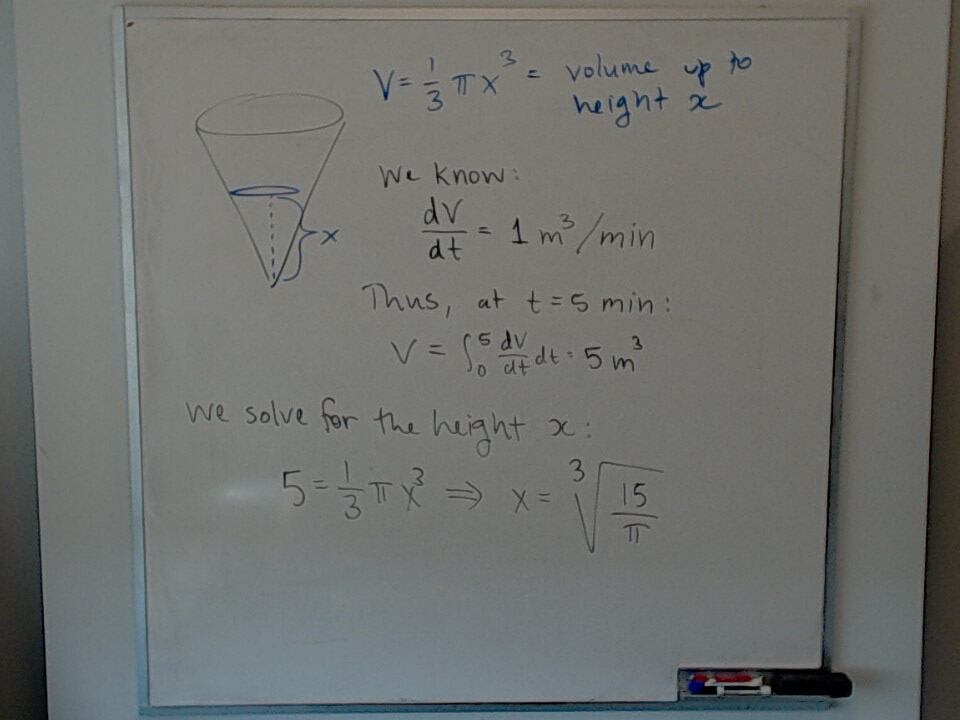# Volume in Conical Tank at t = 5 min

Dec 1, 2022We find the height of water in a conical tank such that:

$$V = \frac{1}{3} \pi x^3$$

given that water is flowing in at a rate $dV/dt = 1 m^3 / min$.

Related tags: